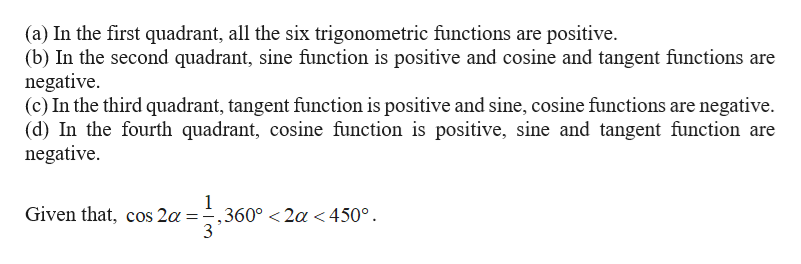# Suppose that cos(2α)=1/3, 360∘<2α<450∘. Find the six trigonometric values of α.

Question
1 views

Suppose that cos(2α)=1/3, 360∘<2α<450∘. Find the six trigonometric values of α.

check_circle

Step 1

Determine the sign of the function values in which of the quadrant as follows.help_outlineImage Transcriptionclose(a) In the first quadrant, all the six trigonometric functions are positive. (b) In the second quadrant, sine function is positive and cosine and tangent functions are negative (c) In the third quadrant, tangent function is positive and sine, cosine functions are negative. (d) In the fourth quadrant, cosine function is positive, sine and tangent function are negative 1 Given that, cos 2a -,360° <2a < 450° 3 fullscreen
Step 2

Find the value of sin α.

Step 3

Find the value of c...

### Want to see the full answer?

See Solution

#### Want to see this answer and more?

Solutions are written by subject experts who are available 24/7. Questions are typically answered within 1 hour.*

See Solution
*Response times may vary by subject and question.
Tagged in
MathTrigonometry

### Trigonometric Ratios